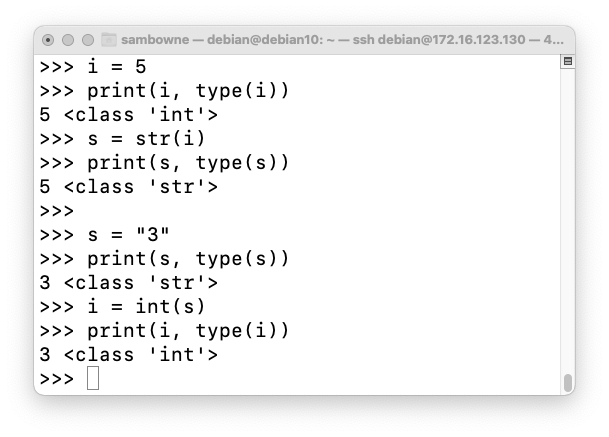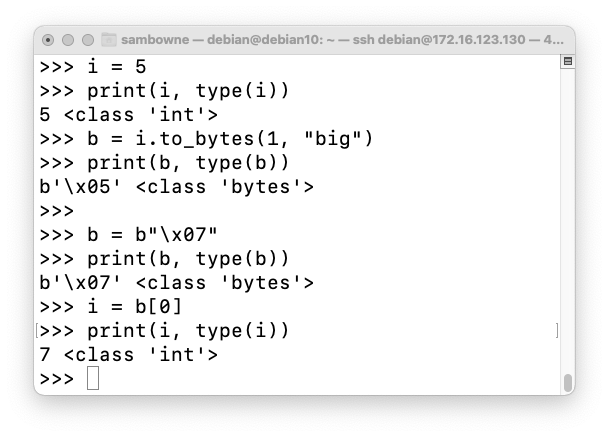# Python3 Conversions

## Integer to/from String

Execute these commands in a Python3 shell:
```i = 5 print(i, type(i)) s = str(i) print(s, type(s)) s = "3" print(s, type(s)) i = int(s) print(i, type(i)) ```
The conversions work, as shown below.## Integer to/from Bytes

Execute these commands in a Python3 shell:
```i = 5 print(i, type(i)) b = i.to_bytes(1, "big") print(b, type(b)) b = b"\x07" print(b, type(b)) i = b print(i, type(i)) ```
The conversions work, as shown below.## String to/from Bytes

Execute these commands in a Python3 shell:
```s = "hello" print(s, type(s)) b = bytes(s, 'utf-8') print(b, type(b)) b = b'HELLO' print(b, type(b)) s = b.decode('utf-8') print(s, type(s)) ```
The conversions work, as shown below.Posted 11-6-21 by Sam Bowne